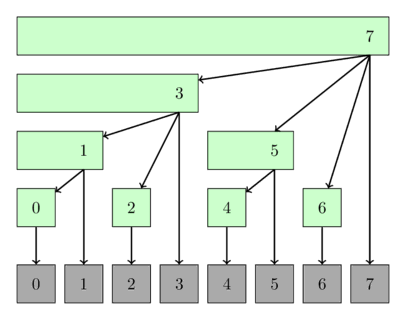# Binary Indexed Tree (Fenwick Tree)

## What is a Binary Indexed Tree

Binary Indexed Tree is a data structure that can store array $A$ of length $n$ with following property

• Calculates the value of function $f$ in the given range $[l, r]$ in $O(log(n))$ time. There are some restriction on which kind of function $f$ you can use, for example sum function works, but min/max function don’t.
• Updates the value of an element of $A$ in $O(log(n))$ time
• Requires only $O(n)$ spaces, which is the input spaces

### Why do we need it

Naive calculation of $f$ in the range $[l,r]$ usually cost $O(n)$. Imagine you have $10^5$ queries, each of them traverse through an array length $10^5$, you would end up having 10 billions traversal and TLE.

## How it works

Here is an example of a BIT representation for an array of 8 items(image taken from cp-algorithms.com)

In this example, let $A$ be the original array and $B$ be the BIT version of $A$. Let $f$ be the sum function for the sake of simplicity.

Here is the process to calculate $f(6) = A_0 + A_1 + A_2 + A_3 + A_4 + A_5 + A_6$

1. Start with $B_6$, $B_6$ covers only $A_6$, we add $B_6$ the the final result
2. Next, we want to go to the next uncovered range, which is $B_{6-1} = B_5$, $B_5$ covers $A[4,5]$, we add $B_5$ to the final result
3. Next uncovered range, $B_{4-1} = B_3$. $B_3$ covers $A[0,3]$. Add $B_3$ to the final result. We end here because we have covered the range $A[0,6]$.

What if you want to calculate $f(5) = A_0 + A_1 + A_2 + A_3 + A_4 + A_5$

1. Start with $B_5$, $B_5$ covers $A[4,5]$, we add $B_5$ to the final result
2. Next, we want to go to the next uncovered range, which is $B_{4-1} = B_3$. $B_3$ covers $A[0,3]$. Add $B_3$ to the final result and we are done.

What if you want to calculate $f(7) = A_0 + A_1 + A_2 + A_3 + A_4 + A_5 + A_6 + A_7$

1. Start with $B_7$, Luckily enough, $B_7$ covers $A[0, 7]$ so we already got the answer, which is $B_7$

### Definition of $g$

The computation of $g(i)$ is defined using the following simple operation: we replace all trailing 1 bits in the binary representation of $i$ with 0 bits.

In other words, if the least significant digit of $i$ in binary is 0, then $g(i)=i$. And otherwise the least significant digit is a 1, and we take this 1 and all other trailing 1s and flip them.

There exists a simple implementation of $g$ using bitwise operator as follow

$g(i) = i\&(i+1)$

### Example of $g$

$g(11) = g(1011_2) = 1000_2 = 8$

It means $B_{11}$ will be covering $A[8,11]$

\begin{align*} g(12) = g(1100_2) = 1100_2 = 12 \\ g(14) = g(1110_2) = 1110_2 = 14 \end{align*}

It means $B_{12}, B_{14}$ will be respectively covering $A_{12}, A_{14}$ only

### C++ implementation

BIT 1D

struct FenwickTree {
vector<int> bit;  // binary indexed tree
int n;

FenwickTree(int n) {
this->n = n;
bit.assign(n, 0);
}

int sum(int r) {
int ret = 0;
for (; r >= 0; r = (r & (r + 1)) - 1)
ret += bit[r];
return ret;
}

int sum(int l, int r) {
return sum(r) - sum(l - 1);
}

void add(int idx, int delta) {
for (; idx < n; idx = idx | (idx + 1))
bit[idx] += delta;
}
};

BIT 2D

struct FenwickTree2D {
vector<vector<int>> bit;
int n, m;

// init(...) { ... }

int sum(int x, int y) {
int ret = 0;
for (int i = x; i >= 0; i = (i & (i + 1)) - 1)
for (int j = y; j >= 0; j = (j & (j + 1)) - 1)
ret += bit[i][j];
return ret;
}

void add(int x, int y, int delta) {
for (int i = x; i < n; i = i | (i + 1))
for (int j = y; j < m; j = j | (j + 1))
bit[i][j] += delta;
}
};